# Eye Dome Lighting¶

Eye-Dome Lighting (EDL) is a non-photorealistic, image-based shading technique designed to improve depth perception in scientific visualization images. To learn more, please see this blog post.

```# sphinx_gallery_thumbnail_number = 1
import pyvista as pv
from pyvista import examples

# Eye dome lighting is not currently supported by VTKjs
pv.rcParams["use_panel"] = False
```

## Statue¶

Eye-Dome Lighting can dramatically improve depth perception when plotting incredibly sophisticated meshes like the creative commons Queen Nefertiti statue:

```nefertiti = examples.download_nefertiti()
nefertiti.plot(eye_dome_lighting=True, cpos=[-1, -1, 0.2], color=True)
```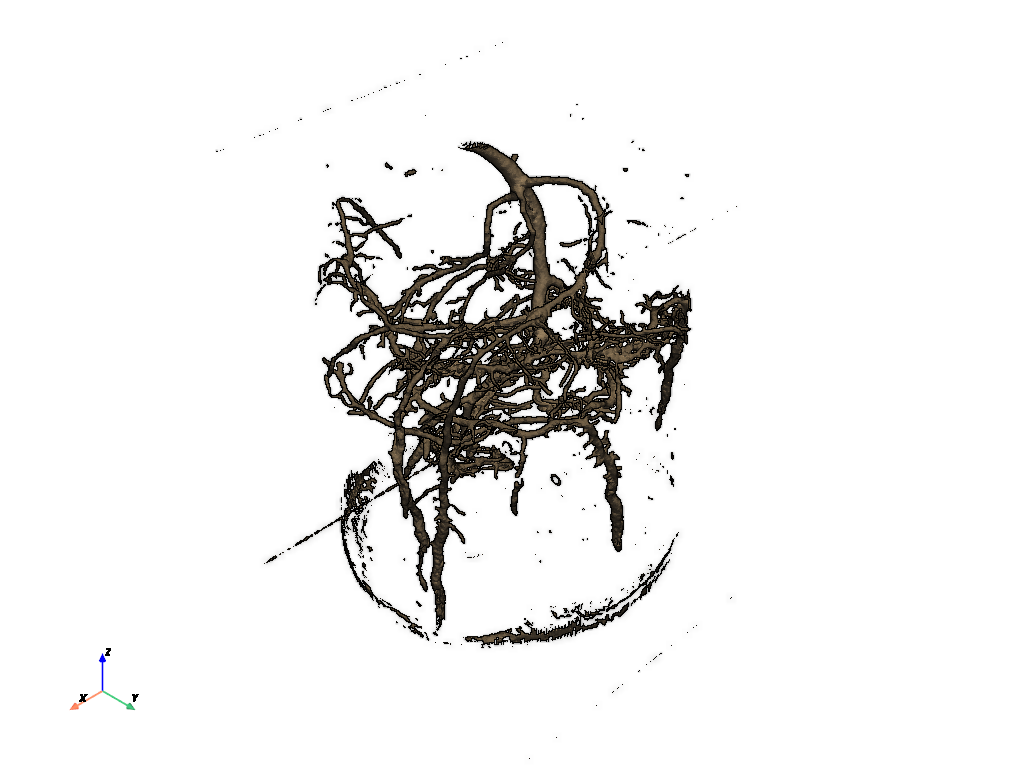Out:

```[(-890.0626454793897, -890.0626454793897, 178.01252909587797), (0.0, 0.0, 0.0), (0.0, 0.0, 1.0)]
```

Here we will compare a EDL shading side by side with normal shading

```p = pv.Plotter(shape=(1, 2), border=False)

# With eye-dome lighting
p.subplot(0, 0)
p.enable_eye_dome_lighting()
p.camera_position = [-1, -1, 0.2]

# No eye-dome lighting
p.subplot(0, 1)
p.camera_position = [-1, -1, 0.2]

p.show()
```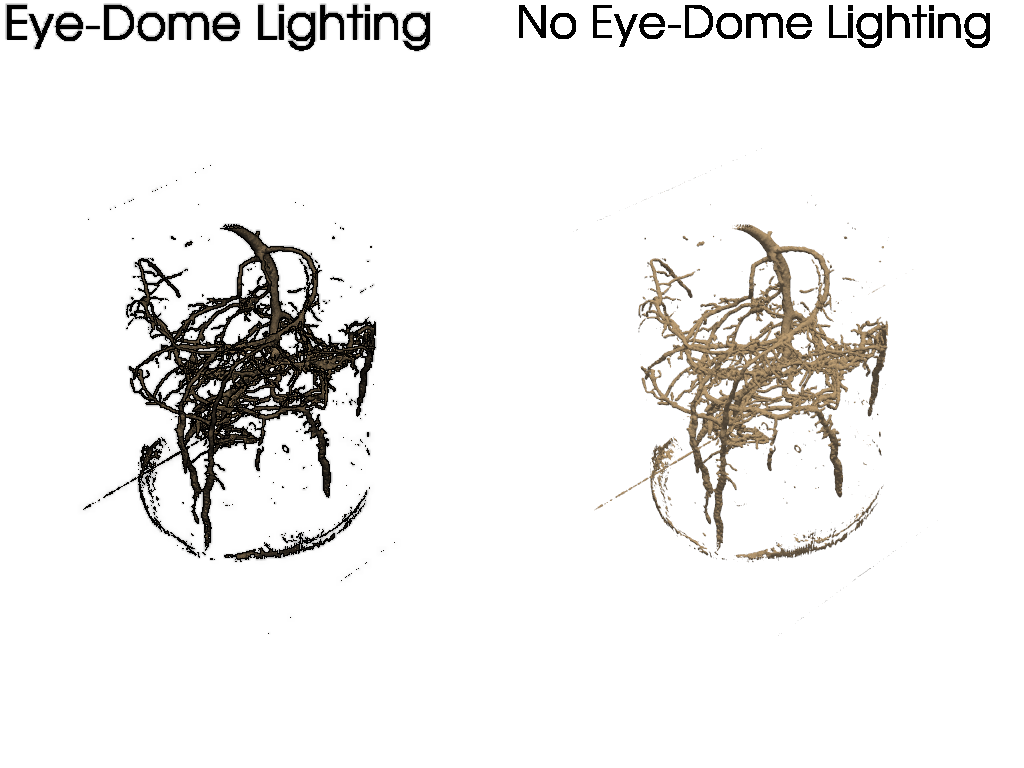Out:

```[(-1310.0155602140887, -1310.0155602140887, 262.0031120428178), (0.0, 0.0, 0.0), (0.0, 0.0, 1.0)]
```

## Point Cloud¶

When plotting a simple point cloud, it can be difficult to perceive depth. Take this Lidar point cloud for example:

```point_cloud = examples.download_lidar()
```

And now plot this point cloud as-is:

```# Plot a typical point cloud with no EDL
p = pv.Plotter()
p.show()
```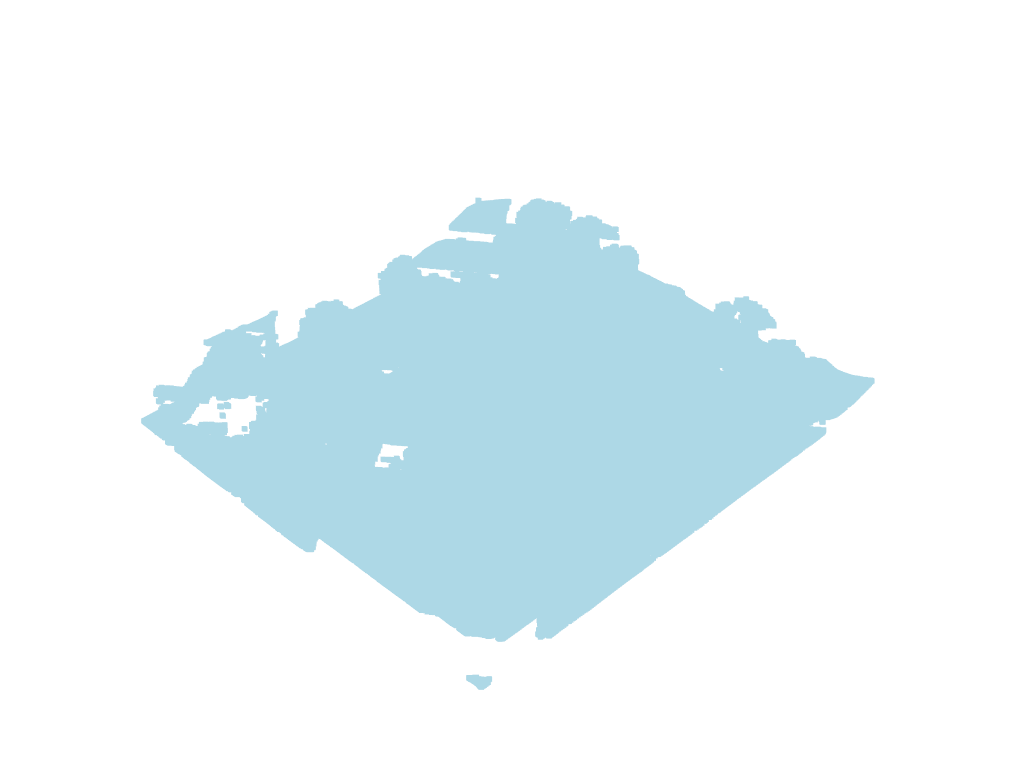Out:

```[(481322.3429432355, 4400455.467942938, 2064.2978993130805), (481028.37499997707, 4400161.49999968, 1770.3299560546875), (0.0, 0.0, 1.0)]
```

We can improve the depth mapping by enabling eye dome lighting on the renderer with `pyvista.Renderer.enable_eye_dome_lighting()`.

```# Plot with EDL
p = pv.Plotter()
p.enable_eye_dome_lighting()
p.show()
```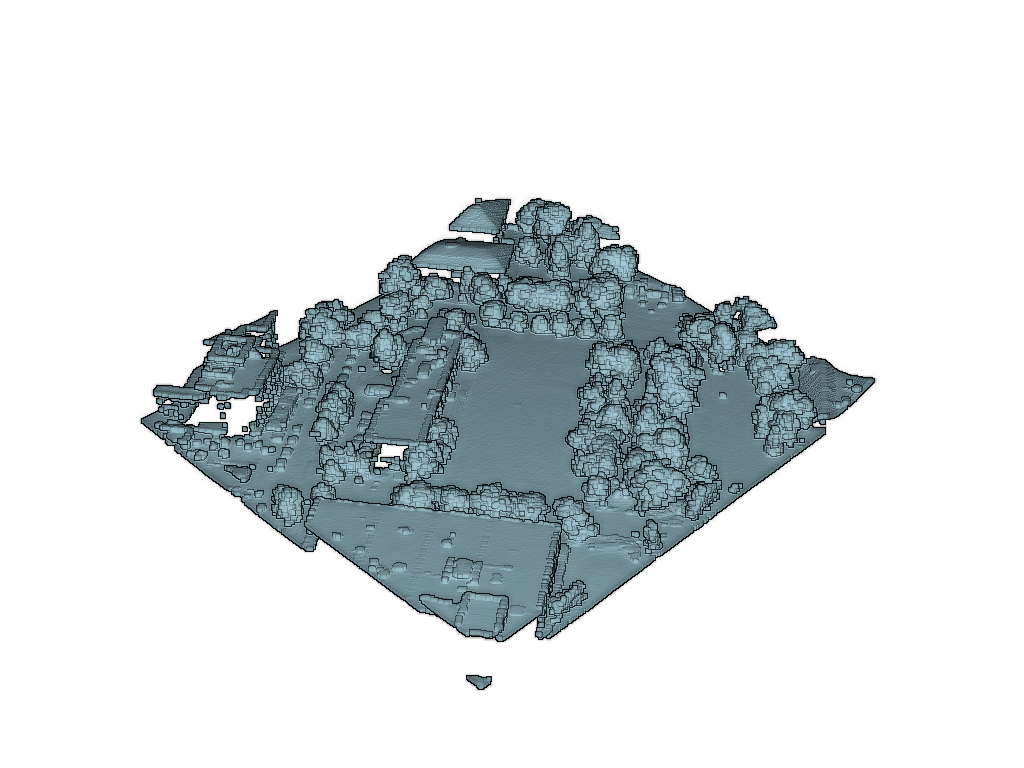Out:

```[(481322.3429432355, 4400455.467942938, 2064.2978993130805), (481028.37499997707, 4400161.49999968, 1770.3299560546875), (0.0, 0.0, 1.0)]
```

The eye dome lighting mode can also handle plotting scalar arrays:

```# Plot with EDL and scalar data
p = pv.Plotter()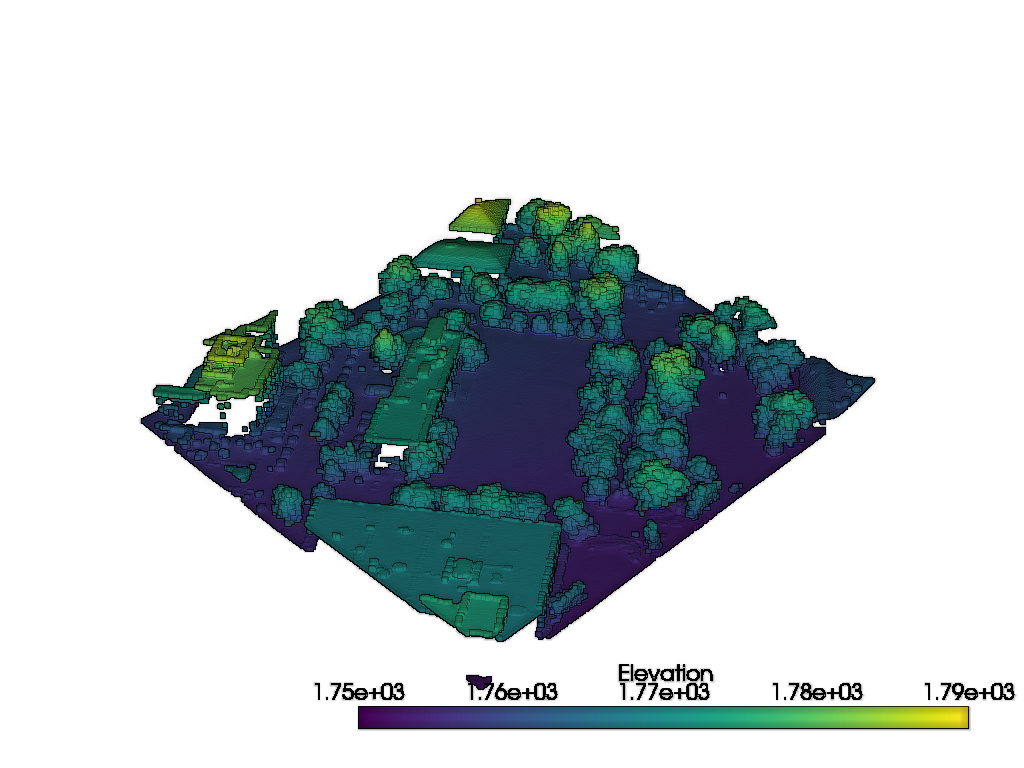```[(481322.3429432355, 4400455.467942938, 2064.2978993130805), (481028.37499997707, 4400161.49999968, 1770.3299560546875), (0.0, 0.0, 1.0)]